Get instant live expert help with Excel or Google Sheets“My Excelchat expert helped me in less than 20 minutes, saving me what would have been 5 hours of work!”

#### Post your problem and you’ll get expert help in seconds.

Your message must be at least 40 characters
Our professional experts are available now. Your privacy is guaranteed.

# Transpose table without zeros

We can use the TRANSPOSE function in combination with the IF Function to flip the orientation of a given range without having the zero snag. The steps below will walk through the process.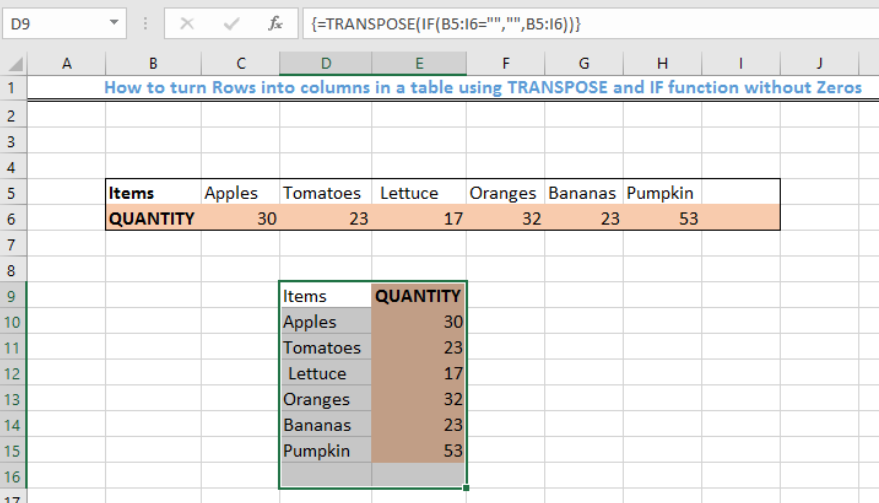Figure 1- How to convert rows to columns in a table without zeros using the TRANSPOSE and IF function

## General Formula

`{=TRANSPOSE(IF(rng="","",rng))}`

## Formula

`=TRANSPOSE(IF(B5:I6="","",B5:I6))`

## Setting up the Data

We will prepare a spreadsheet in a horizontal orientation. Our objective is to use the TRANSPOSE and IF functions to flip the table into a vertical orientation.

• We will type the items in the rows from Cell B5 to Cell H5
• We also type in the quantities for each item in the rows from Cell B6 to Cell H6
• Cell I5 and Cell I6 are left blank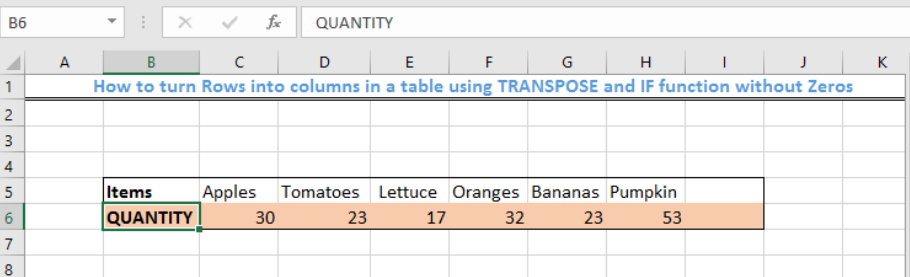Figure 2 – Setting up the Data

## Applying the TRANSPOSE and IF function

• We have 2 rows and 8 columns, therefore, we should highlight 16 cells as shown in figure 3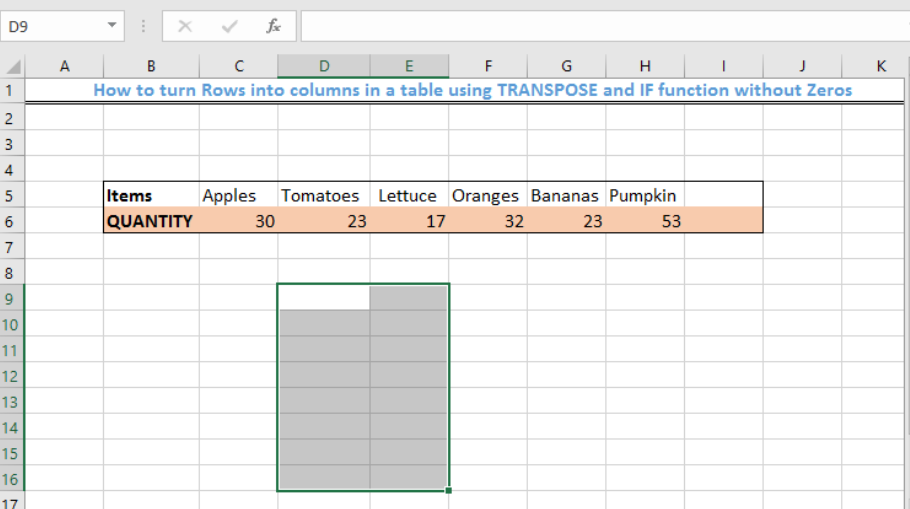Figure 3- Highlighted 16 blank cells

• We will type the formula below into the fx column

`=TRANSPOSE(IF(B5:I6="","",B5:I6))`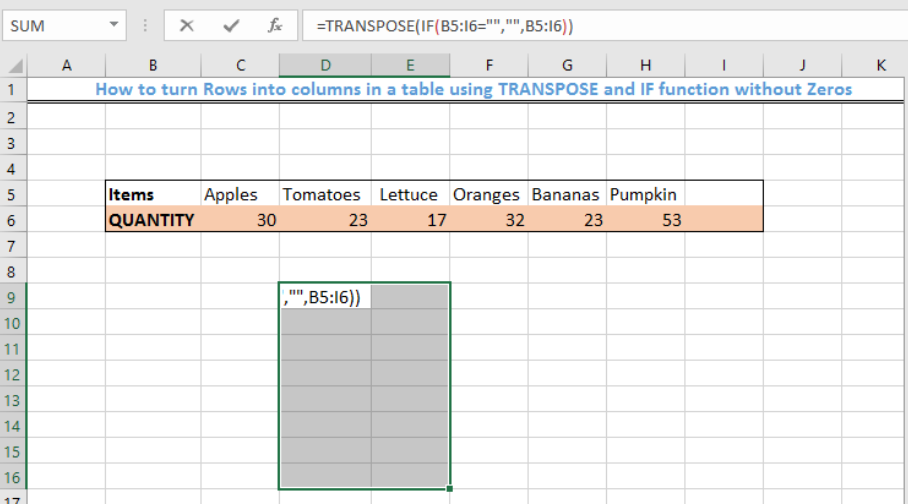Figure 4- Applying the TRANSPOSE and IF Function

• Because this is an array formula, we will PRESS and HOLD the CTRL BUTTON + SHIFT BUTTON + ENTER Key.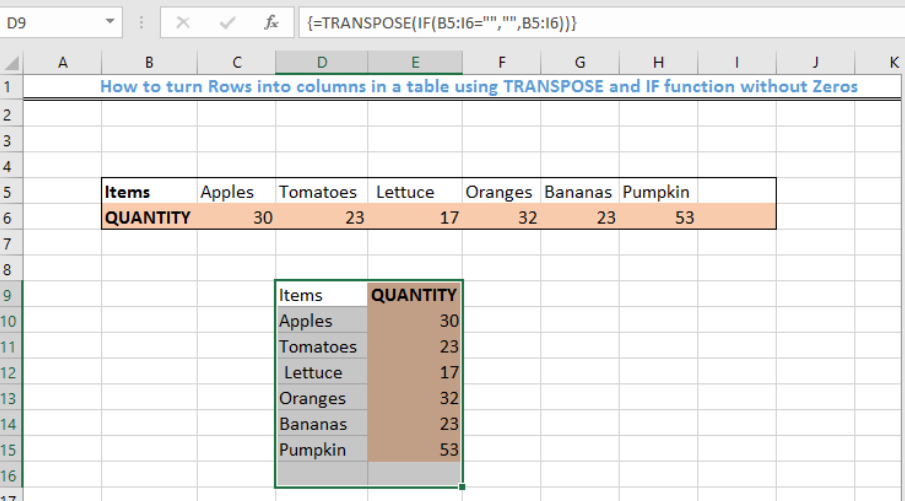Figure 5 – Result of converting rows to column with TRANSPOSE and IF function

## Explanation

`=TRANSPOSE(IF(B5:I6="","",B5:I6))`

• TRANSPOSE function

The TRANSPOSE function changes the orientation of data. If there is an empty or blank cell, TRANSPOSE will “fix” the problem by placing zeros in these cells.

Without the IF Function, the array will look like this:

`{“item”,”apples”,”tomatoes”,lettuce”,”oranges”,”bananas”,”pumpkin”,0;Qty”,30,23,17,32,23,53,0}`

• IF function

The IF function is used to remove the zeros from the blank cells. This is done with this character: =””,””.After applying the IF and TRANSPOSE function, the array will look like this:

`{“item”,”apples”,”tomatoes”,lettuce”,”oranges”,”bananas”,”pumpkin”,””;Qty”,30,23,17,32,23,53,””}`

## Instant Connection to an Expert through our Excelchat Service

Most of the time, the problem you will need to solve will be more complex than a simple application of a formula or function. If you want to save hours of research and frustration, try our live Excelchat service! Our Excel Experts are available 24/7 to answer any Excel question you may have. We guarantee a connection within 30 seconds and a customized solution within 20 minutes.

### Did this post not answer your question? Get a solution from connecting with the expert.Another blog reader asked this question today on Excelchat:
Related blogs
Solution exampleshello i need help creating a formula, if a value does not exsits put 0, if a value exist do nothing
Solved by M. F. in 9 minsCompute the weighted average of students score from a spreadsheet using different weight for each category of marks
Solved by G. E. in 20 minshi there, im making a spread sheet to track progress in a game and would like to create a drop log sheet, i was hoping to use the if function to detect weather a item is logged on a sheet then send the sheet number to a list if so
Solved by K. F. in 60 minsI have a schedule I'm doing with formulas to add workdays (across rows) to a start date column. I would like to write the equation like this: =ifError((workday(b2,1) = cell above, [value of cell above + 1] Help me form this correctly to get the (value of target cell) = (1 more than the value from the cell above) IF the target cell value started equal to the cell above.
Solved by M. J. in 20 minsI need a formula that will subtract the sum of one cell if the sum of another cell is greater than zero, and have it applied to cells across a document that is 30,000 entries and only in certain spots
Solved by F. J. in 20 mins## Subscribe to Excelchat.coAnother blog reader asked this question today on Excelchat: